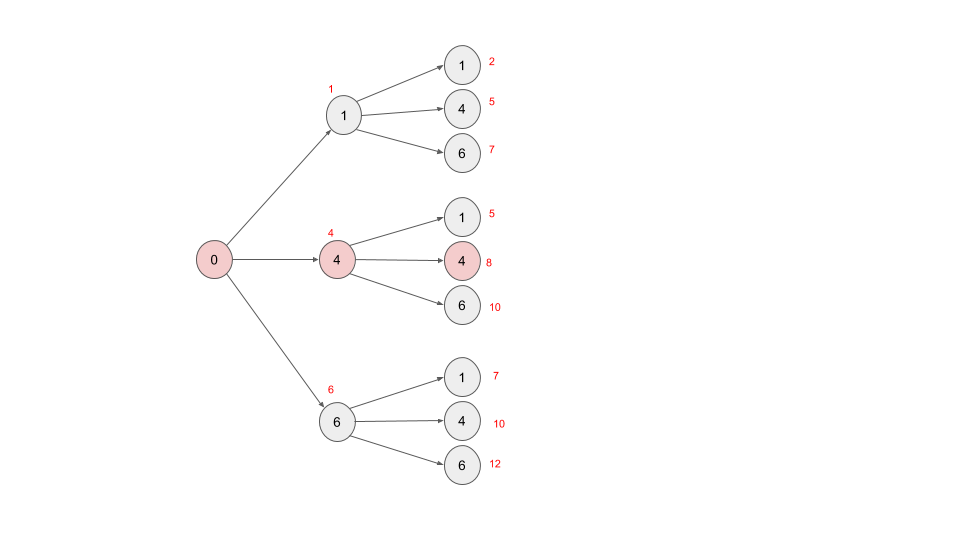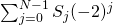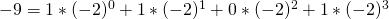# The Power of Generic Algorithms

### Learn how generic algorithms can help you better understand problems in different domains, and how this can help you optimize performance.

· Performance Zone · Tutorial
Save
6.73K Views

in computer science, there is this tension between creating generic algorithms applicable to different situations and optimizing an algorithm to solve a specific problem.

generic algorithms make it possible to find similarities between problems that, at first glance, may seem completely unrelated. this is especially interesting when those problems belong to different domains. when this happens, the nature of the problem is better understood and ideas from one domain can be transferred to another.

however, algorithms need to be executed in physical machines, and therefore limitations, of time and space must be taken into account. unfortunately, many optimized algorithms have little resemblance to its generic version. in a way, information is lost when moving from a generic algorithm to its optimized version.

the aim of this post is to illustrate the use of a generic algorithm to solve problems as disparate as:

• chess knight problem : given a chessboard, find the shortest distance (minimum number of steps) to move a knight from a square to another.
• problem of the knight's tour : find the sequence of moves of a knight on a chessboard such that the knight visits every square only once
• minimum coin change : find the minimum number of coins (of certain denominations) that add up to a given amount of money
• representation of an integer in base -2 : instead of the normal binary base (2), it's possible to use negative bases like -2

## graph representation

in order to solve these problems, we will use a common representation for all of them, namely: a graph. therefore, the first step will be to define a way to traverse the graph.

``````trait graphtraversal[t] {

type vertex = t
//path to a vertex
type path = list[vertex]

def findpath(from: seq[path]): path = {

val paths: listbuffer[path] = listbuffer() ++= from

def next(): path = paths.headoption match{
case none => nil
case some(currentvertexpath) =>
if(issolution(currentvertexpath)) currentvertexpath.reverse
else {
val currentvertex = currentvertexpath.head
val neighbourvertices = neighbours(currentvertex).filter(isvertexeligibleforpath(_, currentvertexpath))
val pathstoneighbourvertices = neighbourvertices.map(_ :: currentvertexpath)
paths.remove(0)
addneighbours(paths, pathstoneighbourvertices)
next()
}
}
next()
}

def neighbours(vertex: vertex): seq[vertex]
def addneighbours(verticestoexplore: listbuffer[path], neighbours: seq[path]): unit
def issolution(path: path): boolean
def isvertexeligibleforpath(vertex: vertex, path: path): boolean``````

the method ``` findpath ``` , starting at the vertices ``` from ``` , traverses the graph and as it does so, builds all possible paths until finding the solution as per the definition of the method ``` issolution ``` . this method defines the condition to be met by the path, e.g. the path must pass through an specific vertex or through all the vertices.

the process to calculate the neighbours of a given vertex depends on the topology ("shape") of the graph and is implemented by the method ``` neighbours ``` .

the method ``` isvertexeligibleforpath ``` filters the neighbours that are eligible to be included in a path (for instance, in most cases it is desirable not to visit twice the same vertex and consequently vertices already visited are discarded).

the method ``` addneighbours ``` adds the neighbours of the current vertex to the list of remaining vertices to explore and determines the way the graph is traversed: depth-first or breadth-first (depending on whether the new vertices are added at the front or at the back of the list, respectively)

again, i cannot stress enough that the implementation of ``` findpath ``` is very generic and therefore sub-optimal for the problems mentioned above.

the following sections show how to solve said problems.

before getting started, here are some definitions that will be needed later on:

``````type coin = int
type coordinate = (int, int)

implicit class richtuple2(coordinate: coordinate){
def +(other: coordinate) = (coordinate._1 + other._1, coordinate._2 + other._2)
def -(other: coordinate) = (coordinate._1 - other._1, coordinate._2 - other._2)
}``````

## chess knight problem

probably, this is the most intuitive of the problems as it is easy to imagine the chessboard like a graph where the squares are the vertices that are connected by the moves of the knight. with this in mind, we can represent an infinite chessboard like so:

``````trait infinitechessboard extends graphtraversal[coordinate]{

//knight moves
val moves: list[coordinate] = list((2,1), (1,2), (-1,2), (-2,1), (-2,-1), (-1,-2), (1,-2), (2,-1))

override def neighbours(coordinate: coordinate): seq[coordinate] = moves.map( coordinate + _)

/**
* the shortest path cannot include the same vertex twice
*/
override def isvertexeligibleforpath(vertex: coordinate, path: path): boolean = !path.contains(vertex)
}``````

the remaining methods are defined in another trait describing the nature of the problem:

• the method ``` addneighbours ``` must implement a breadth-first search in order to find the shortest path
• the method ``` issolution ``` ensures that the solution path contains the destination
``````trait shortestpath extends graphtraversal[coordinate]{

self: destination[coordinate] =>

override def addneighbours(verticestoexplore: listbuffer[path], neighbours: seq[path]): unit =
verticestoexplore ++= neighbours

override def issolution(path: path): boolean = path.head == to
}

trait destination[t]{
def to(): t
}``````

finally, the class implementing the solution can be defined by stacking all these traits:

``case class shortestpathininfiniteboard(to: coordinate) extends infinitechessboard with shortestpath with destination[coordinate]``

example:

``````test("shortest path between (0,0) -> (3,3)"){
val from = (0,0)
val to = (3,3)
val solution = list((0,0), (2,1), (3,3))
assert(shortestpathininfiniteboardapp(to).findpath(list(list(from))) == solution)
}``````

## knight's tour

the first thing to consider here is that the chessboard must be finite, what can be achieved by overwriting the trait ``` infinitechessboard ``` to restrict the moves of the knight to the dimensions of the board. the original implementation of ``` isvertexeligibleforpath ``` is still valid as the knight's path cannot visit the same square twice.

``````trait finitechessboard extends infinitechessboard{

self: boarddim =>

override def neighbours(coordinate: coordinate): seq[coordinate] =
super.neighbours(coordinate).filter{ case (v, w) => v >= 0 && v<x && w >=0 && w<y }
}

trait boarddim{
def x(): int
def y(): int
}``````

the nature of the problem is represented by the following trait:

• the traversal of the graph is done in a depth-first fashion
• the solution is the path that visits all vertices
``````trait knighttour extends graphtraversal[coordinate]{

self: finitechessboard with boarddim =>

override def addneighbours(verticestoexplore: listbuffer[path], neighbours: seq[path]): unit =
verticestoexplore.prependall(neighbours) //depth-first search

override def issolution(path: path): boolean = path.length == x * y
}``````

finally, the solution is

``case class knighttourinfiniteboard(x: int, y: int) extends knighttour with finitechessboard with boarddim``

example:

``````test("tour in a board of dimensions (8,8) starting at (0,0)"){
val dim = (8,8)
val from = (0,0)
val solution = list((0,0), (2,1), (4,2), (6,3), (7,5), (6,7), (4,6), (2,7), (0,6), (1,4), (3,5), (5,6), (7,7), (6,5), (5,7), (3,6), (1,7), (0,5), (2,6), (4,7), (5,5), (7,6), (6,4), (4,5), (6,6), (5,4), (3,3), (2,5), (3,7), (1,6), (0,4), (1,2), (2,4), (0,3), (1,1), (3,0), (2,2), (1,0), (0,2), (2,3), (4,4), (3,2), (4,0), (6,1), (7,3), (5,2), (7,1), (5,0), (3,1), (4,3), (5,1), (7,0), (6,2), (7,4), (5,3), (7,2), (6,0), (4,1), (2,0), (0,1), (1,3), (3,4), (1,5), (0,7))
assert(knighttourinfiniteboardapp(dim._1, dim._2).findpath(list(list(from))) == solution)
}``````

## minimum coin change problem

surprisingly, or maybe not if you are familiar with this problem, the optimal change can be represented as a graph. each vertex represents the face value of a coin and the solution is a path such that the sum of its vertices equals the original amount. for instance, for a set of coins [1,4,6], the amount 8 is represented by the path 0 -> 4 -> 4.optimal change graph representation.

after the picture, it's easy to implement graphtraversal

``````trait optimalchange extends graphtraversal[coin]{

self: coins with amount =>

val moves: list[coin] = coins

override def neighbours(vertex: int): seq[vertex] = moves

/**
* the nature of the problem requires a breadth-first search in order to find the shortest path (minimum number of coins)
*/
override def addneighbours(verticestoexplore: listbuffer[path], neighbours: seq[path]): unit =
verticestoexplore ++= neighbours

override def issolution(path: path): boolean = amount == path.sum

override def isvertexeligibleforpath(vertex: int, path: path): boolean = (amount - path.sum) >= vertex
}

trait coins {
def coins(): list[coin]
}

trait amount {
def amount(): int
}``````

and the solution is

``````class optimalchangeapp(val coins: list[coin], val amount: int) extends optimalchange with coins with amount{
def optimalchange() = findpath(list(list(0))) match{
case nil => nil
case xs => xs.tail //to remove the first 0
}
}``````

example:

``````test("change of 8 with set of coins (1,4,6)"){
assert(optimalchangeapp(list(1,4,6),8).optimalchange() == list(4,4))
}``````

## representation of an integer in base -2

in base -2, integers are represented by sequences of bits in the following way. sequence s of n bits represents the numberfor instance,negative binary base graph representation.

``````trait negativebinary extends graphtraversal[int]{

self: amount =>

val moves: list[int] = list(0, 1)

override def neighbours(vertex: vertex): seq[vertex] = moves

override def addneighbours(verticestoexplore: listbuffer[path], neighbours: seq[path]): unit =
verticestoexplore ++= neighbours

override def issolution(path: path): boolean =
path.reverse.zipwithindex.foldleft(0){case (acc, (vertex, idx)) => acc + vertex * bigint(-2).pow(idx).toint} == amount

override def isvertexeligibleforpath(vertex: vertex, path: path): boolean = true
}

trait amount {
def amount(): int
}``````

and the solution is

``````class negativebinaryapp(val amount: int) extends negativebinary with amount{

def findshortestbinaryrepresentation() = findpath(list(list(0),list(1)))
}``````

example:

``````test("-9 == {1,1,0,1}"){
val number = -9
assert(negativebinaryapp(number).findshortestbinaryrepresentation() == list(1,1,0,1))
}``````

the code of all the examples presented in this post can be found on https://github.com/falvarezb/blog-bytecode/tree/graphs .

Topics:
performance, performance optimization, algorithms

Published at DZone with permission of Francisco Alvarez, DZone MVB.

Opinions expressed by DZone contributors are their own.

Comments